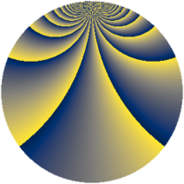# Properties

 Label 2880.2.cyLevel $2880$ Weight $2$ Character orbit 2880.cy Rep. character $\chi_{2880}(223,\cdot)$ Character field $\Q(\zeta_{12})$ Dimension $576$ Sturm bound $1152$

# Learn more about

## Defining parameters

 Level: $$N$$ $$=$$ $$2880 = 2^{6} \cdot 3^{2} \cdot 5$$ Weight: $$k$$ $$=$$ $$2$$ Character orbit: $$[\chi]$$ $$=$$ 2880.cy (of order $$12$$ and degree $$4$$) Character conductor: $$\operatorname{cond}(\chi)$$ $$=$$ $$360$$ Character field: $$\Q(\zeta_{12})$$ Sturm bound: $$1152$$

## Dimensions

The following table gives the dimensions of various subspaces of $$M_{2}(2880, [\chi])$$.

Total New Old
Modular forms 2400 576 1824
Cusp forms 2208 576 1632
Eisenstein series 192 0 192

## Trace form

 $$576q + O(q^{10})$$ $$576q + 96q^{81} + O(q^{100})$$

## Decomposition of $$S_{2}^{\mathrm{new}}(2880, [\chi])$$ into newform subspaces

The newforms in this space have not yet been added to the LMFDB.

## Decomposition of $$S_{2}^{\mathrm{old}}(2880, [\chi])$$ into lower level spaces

$$S_{2}^{\mathrm{old}}(2880, [\chi]) \cong$$ $$S_{2}^{\mathrm{new}}(360, [\chi])$$$$^{\oplus 4}$$$$\oplus$$$$S_{2}^{\mathrm{new}}(1440, [\chi])$$$$^{\oplus 2}$$